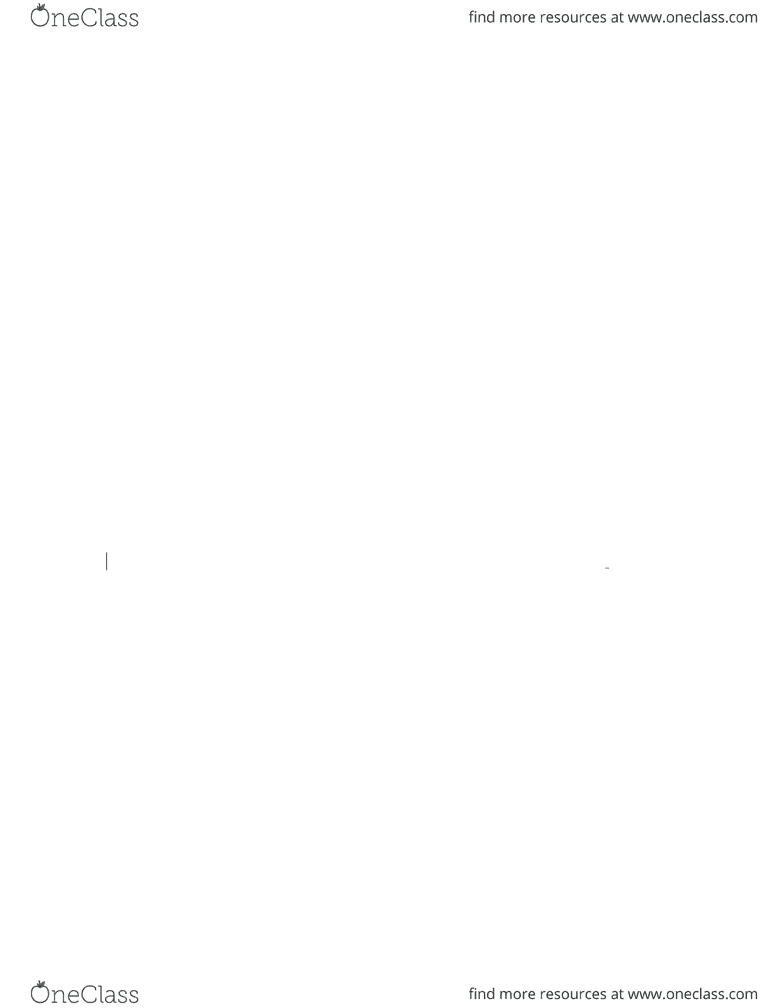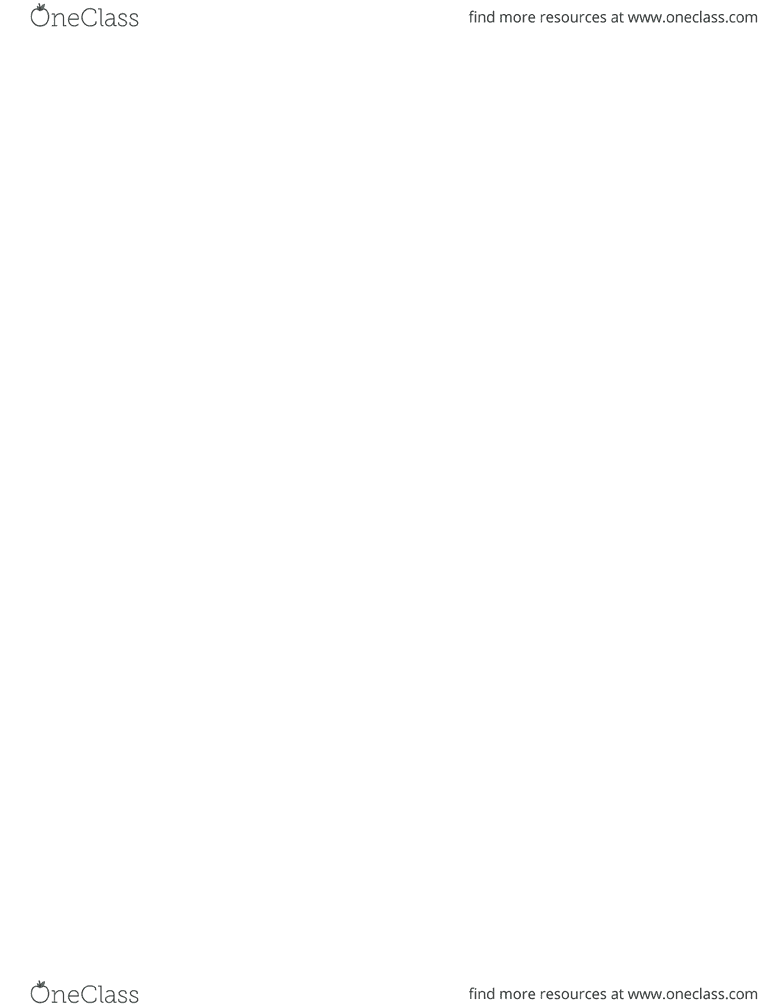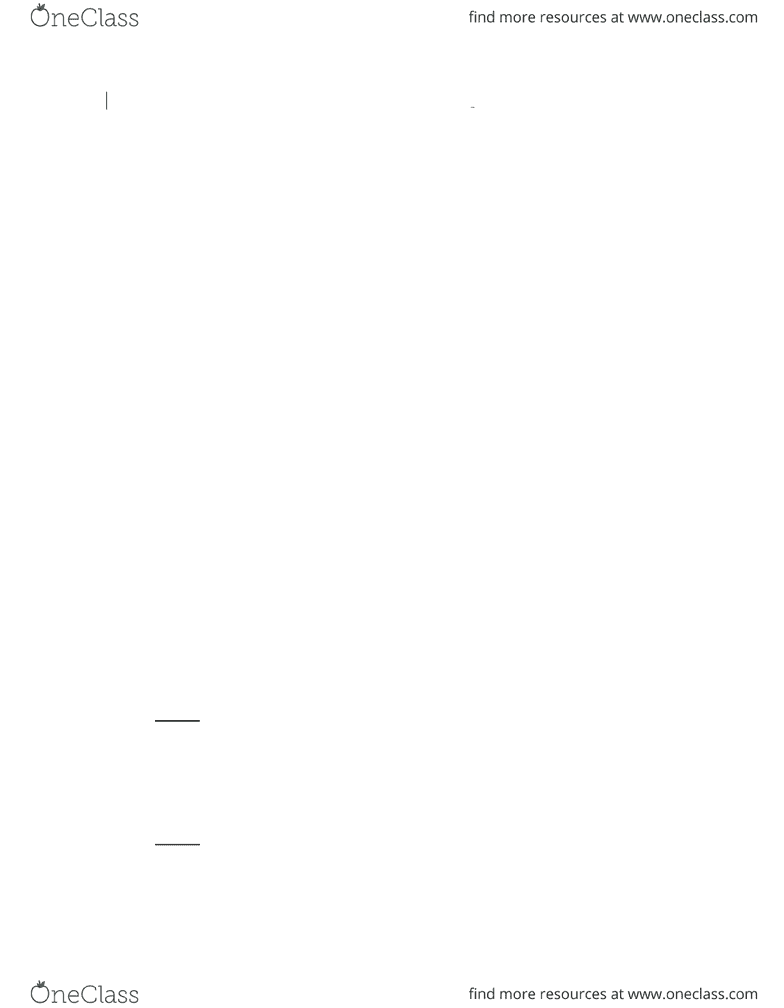Textbook Notes (270,000)
CA (160,000)
York (10,000)
ADMS (1,000)
Chapter 6

# ADMS 3530 Chapter Notes - Chapter 6: Subordinated Debt, Risk Premium, Liquidity Risk

Department
Administrative Studies
Course Code
ADMS 3530
Professor
Lois King
Chapter
6

This preview shows pages 1-3. to view the full 21 pages of the document.Brealey 5CE
Solutions to Chapter 6
Note: Unless otherwise stated, assume all bonds have \$1,000 face (par) value.
1. a. The coupon payments are fixed at \$60 per year.
Coupon rate = coupon payment/par value = 60/1000 = 6%, which remains
unchanged.
b. When the market yield increases, the bond price will fall. The cash flows are
discounted at a higher rate.
c. At a lower price, the bond’s yield to maturity will be higher. The higher
yield to maturity on the bond is commensurate with the higher yields
available in the rest of the bond market.
d. Current yield = coupon payment/bond price. As coupon payment remains the
same and the bond price decreases, the current yield increases.
2. When the bond is selling at a discount, \$970 in this case, the yield to maturity is
greater than 8%. We know that if the discount rate were 8%, the bond would sell at par.
At a price below par, the YTM must exceed the coupon rate.
Current yield equals coupon payment/bond price, in this case, 80/970. So, current yield
is also greater than 8%.
3. Coupon payment = .08 x 1000 = \$80
Current yield = 80/bond price = .07
Therefore, bond price = 80/.07 = \$1,142.86
4. Par value is \$1000 by assumption.
Coupon rate = \$80/\$1000 = .08 = 8%
Current yield = \$80/\$950 = .0842 = 8.42%
Yield to maturity = 9.1185%
[Enter in the calculator: N = 6; PV= -950; FV = 1000; PMT = 80]
5. To sell at par, the coupon rate must equal yield to maturity. Since Circular bonds
yield 9.1185%, this must be the coupon rate.
5-1
Copyright © 2006 McGraw-Hill Ryerson Limited

Only pages 1-3 are available for preview. Some parts have been intentionally blurred.5-2
Copyright © 2006 McGraw-Hill Ryerson Limited

Only pages 1-3 are available for preview. Some parts have been intentionally blurred.6. a. Current yield = annual coupon/price = \$80/1,100 = .0727 = 7.27%.
b. On the calculator, enter PV = -1100, FV = 1000, n = 10, PMT = 80
Then compute I/Y (or i) and will get YTM = 6.6023%.
7. When the bond is selling at par, its yield to maturity equals its coupon rate. This
firm’s bonds are selling at a yield to maturity of 9.25%. So the coupon rate on the
new bonds must be 9.25% if they are to sell at par.
8. The current bid yield on the bond was 3.09%. To buy the bond, investors pay the
ask price. The investor would pay 108.21 percent of par value. With \$1,000 par
value, this means paying \$1,082.1 to buy a bond.
9. Coupon payment = interest = .05 × 1000 = 50
Capital gain = 1100 – 1000 = 100
Rate of return = = = .15 = 15%
10. Tax on interest received = tax rate × interest = .3 × 50 = 15
After-tax interest received = interest – tax = 50 – 15 = 35
Fast way to calculate:
After-tax interest received = (1 – tax rate) × interest = (1 – .3)× 50 = 35
Tax on capital gain = .5 × .3 × 100 = 15
After-tax capital gain = 100 – 15 = 85
Fast way to calculate:
After-tax capital gain = (1 – tax rate) × capital gain = (1 – .5×.3)×100 = 85
After-tax rate of return =
= = .12 = 12%
11. Bond 1
year 1: PMT = 80, FV = 1000, i = 10%, n = 10; Compute PV0 = \$877.11
year 2: PMT = 80, FV = l000, i = 10%, n = 9; Compute PV1 = \$884.82
Rate of return = = .10 = 10%
Bond 2
year 1: PMT = 120, FV = 1000, i = 10%, n = 10; Compute PV0 = \$1122.89
year 2: PMT = 120, FV = l000, i = 10%, n = 9; Compute PV1 =\$1115.18
5-3
Copyright © 2006 McGraw-Hill Ryerson Limited
###### You're Reading a Preview

Unlock to view full version